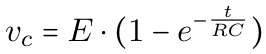# Capacitor charging graph web app in JS

- 2 mins

Recently, I faced the task of analyzing the graph of a capacitor in a simple circuit configuration: a voltage source powering the given capacitor connected to a resistor in series.

The result of this configuration is pretty straightforward: my capacitor will get charged with a certain value of voltage from the source (almost 100%), respecting the exponential function described below:We can easily see that it corresponds to a time versus voltage (exponential) function, where the RC element is what we call time constant. If you multiply the value of your capacitance with the value of the resistance that you have configured in your circuit, the result will represent how long it took for your capacitor to get charged with approximately 63% of the voltage from the source.

As an example, let’s suppose we have a 12V source powering a 1000uF capacitor connected to a 51k resistor: it would take 51 seconds for the capacitor to have stored approximately 7.5V between its terminals.

### Solving the problem: Javascript + Google API

As I didn’t have an oscilloscope, an analog port from a microcontroller board, nor any other resource that would help me to see the graph with the values of time and voltages plotted instantly, the first idea was to use the timer from my cellphone, marking laps and then after plotting the graph on another specific software.

Thus, I thought that it would be really nice to have an application where I could put it all together (timer, time constant calculation and plotted graph) in one single page. The hassle of switching between my phone and my circuit, having to write down the values of times, made me think of this solution that I called Cap-Charge.

### First version and demonstration video

Although I think it would be better to work on all the single bugs and put the almost perfect solution, I decided to put online a very first version (0.1) so as other people use it, we can keep improving it together. I also recorded a video for those who are interested in using it and seeing how it works (Link is here).

I’ve also realized that the application can be very helpful to use in lab experiments in general, so I hope it optimizes your time whether you’re doing it in school or at home. Please let me know if you have any questions. Have fun!